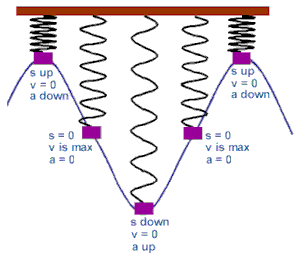# simple harmonic motion

## 3 basic equationsThe diagram shows the relationship between the spring actions and the sine wave.

## derivations of simple harmonic motion equations

The displacement of a body performing simple harmonic motion is described by the following equation,                                                                         x = A sin (ωt + φ) where A is the amplitude,           ω is the natural frequency and           φ is the phase angle. Given A = 35 mm, ω= 50 rad/s and φ = π/8 radian, calculate the acceleration (in mm/s2) when t = T/4.